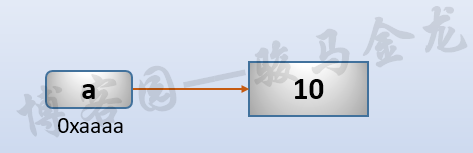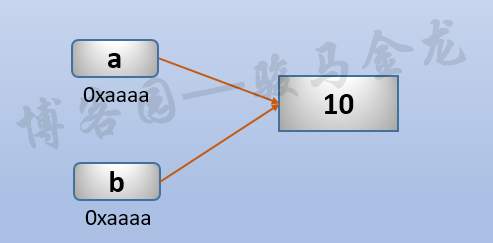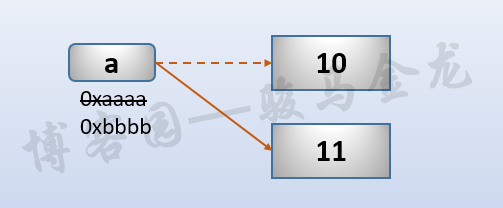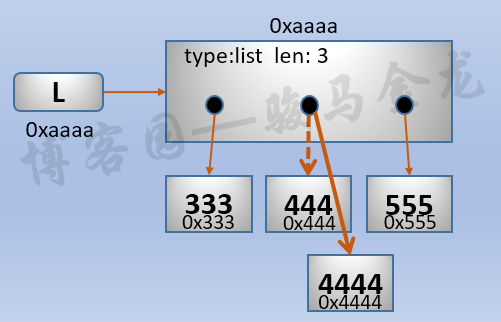# python按引用赋值和深、浅拷贝

## 按引用赋值而不是拷贝副本

### 按引用赋值的特性

a = 10000
b = a

>>> a,b
(10000, 10000)


a = 10000
b = 10000


a = 10000
b = a
a = 20000

>>> a,b
(20000, 10000)


L1 = [111,222,333]
L2 = L1
L1 = 2222

>>> L1,L2
([111, 2222, 333], [111, 2222, 333])


L1赋值的前后，数据对象[111,222,333]的地址一直都没有改变，但是这个列表的第二个元素的值已经改变了。因为L1和L2都指向这个列表，所以L1修改第二个元素后，L2的值也相应地到影响。也就是说，L1和L2仍然是同一个列表对象[111,2222,333]

### 按引用赋值的过程分析>>> L=[333,444,555]
>>> id(L),id(L)
(56583832, 55771984)
>>> L=4444
>>> id(L),id(L)
(56583832, 55771952)## 早就存在的小整数

>>> a=2
>>> b=2
>>> a is b
True


>>> sys.getrefcount(2)
78
>>> a=2
>>> sys.getrefcount(2)
79


>>> a=20000
>>> b=20000
>>> a is b
False


>>> a=20000;b=20000
>>> a is b
True
>>> a,b=20000,20000
>>> a is b
True


a = 25700
b = 25700
print(a is b)      # True

def f():
c = 25700
d = 25700
print(c is d)  # True
print(a is c)  # False

f()


## 深拷贝和浅拷贝

L1 = [1,2,3]
L2 = L1


L1 = [1,[2,22,222],3]
L2 = L1


• 浅拷贝：shallow copy，只拷贝第一层的数据。python中赋值操作或copy模块的copy()就是浅拷贝
• 深拷贝：deep copy，递归拷贝所有层次的数据，python中copy模块的deepcopy()是深拷贝

L = [2,22,222]
L1 = [1,2,3]
L2 = [1,L,3]


L和L1都只有一层深度，L2有两层深度。浅拷贝时只拷贝第一层的数据作为副本，深拷贝递归拷贝所有层次的数据作为副本。

>>> L=[2,22,222]
>>> L1=[1,L,3]
>>> L11 = copy.copy(L1)

>>> L11,L1
([1, [2, 22, 222], 3], [1, [2, 22, 222], 3])

>>> L11 is L1
False
>>> id(L1),id(L11)         # 不相等
(17788040, 17786760)
>>> id(L1),id(L11)   # 相等
(17787880, 17787880)


>>> L111 = copy.deepcopy(L1)

>>> L1,L111
([2, 22, 222], [2, 22, 222])

>>> id(L1),id(L111)
(17787880, 17787800)


>>> s = [1,2,[3,33,333,3333]]
>>> s1 = copy.copy(s)

>>> s1 = 333333333

>>> s, s1
([3, 33, 333, 333333333], [3, 33, 333, 333333333])


posted @ 2018-12-15 13:44  骏马金龙  阅读(6101)  评论(4编辑  收藏  举报